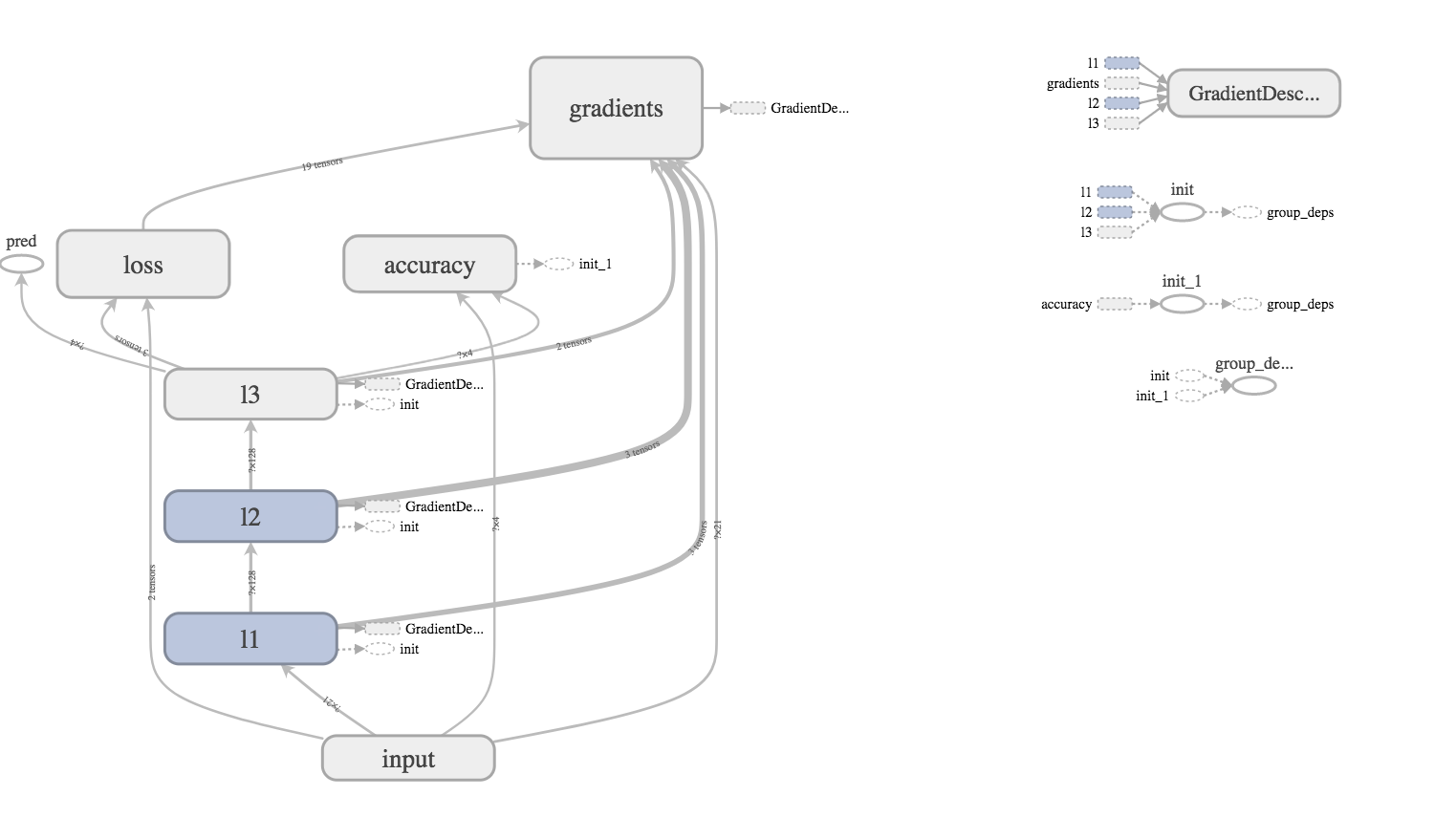# 从头开始做一个汽车状态分类器2: 搭建模型

## 导入数据 ¶

import numpy as np
import tensorflow as tf
import matplotlib.pyplot as plt
import data_processing

new_data = data_processing.convert2onehot(data)


# prepare training data
new_data = new_data.values.astype(np.float32)       # change to numpy array and float32
np.random.shuffle(new_data)
sep = int(0.7*len(new_data))
train_data = new_data[:sep]                         # training data (70%)
test_data = new_data[sep:]                          # test data (30%)


## 搭建网络 ¶

# build network
tf_input = tf.placeholder(tf.float32, [None, 25], "input")
tfx = tf_input[:, :21]
tfy = tf_input[:, 21:]

l1 = tf.layers.dense(tfx, 128, tf.nn.relu, name="l1")
l2 = tf.layers.dense(l1, 128, tf.nn.relu, name="l2")
out = tf.layers.dense(l2, 4, name="l3")
prediction = tf.nn.softmax(out, name="pred")

loss = tf.losses.softmax_cross_entropy(onehot_labels=tfy, logits=out)
accuracy = tf.metrics.accuracy(          # return (acc, update_op), and create 2 local variables
labels=tf.argmax(tfy, axis=1), predictions=tf.argmax(out, axis=1),)
train_op = opt.minimize(loss)

sess = tf.Session()
sess.run(tf.group(tf.global_variables_initializer(), tf.local_variables_initializer()))## 训练网络 ¶

for t in range(4000):
# training
batch_index = np.random.randint(len(train_data), size=32)
sess.run(train_op, {tf_input: train_data[batch_index]})

if t % 50 == 0:
# testing
acc_, pred_, loss_ = sess.run([accuracy, prediction, loss], {tf_input: test_data})
print("Step: %i" % t,"| Accurate: %.2f" % acc_,"| Loss: %.2f" % loss_,)